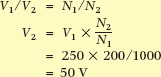Home / Transformer / Transformation Ratio of Transformer

Transformation Ratio of Transformer

Want create site? Find Free WordPress Themes and plugins.

This article covers various transformation ratios of a transformer such as voltage (turns) ratio, current ratio, and impedance ratio (matching) in detail along with solved examples.Voltage (Turns) Ratio

The mutual flux is common to each winding. Therefore, it must induce the same voltage per turn in each winding. If V1 is the total induced voltage in the primary winding having N1 turns, then the induced voltage per turn is V1/N1. Similarly, the induced voltage per turn in the secondary winding is V2/N2.

On no load, the applied voltage V1 and the self-induced voltage V1 are almost equal and V2 = V2, so the above ratios are transposed and usually expressed as:

$\frac{{{V}_{1}}}{{{V}_{2}}}=\frac{{{N}_{1}}}{{{N}_{2}}}$

That is, on no load, the ratio of the voltages is equal to the ratio of the turns.

Turns Ratio Example

A transformer has 1000 turns on the primary winding and 200 on the secondary. If the applied voltage is 250 V, calculate the output voltage of the transformer.Current Ratio

When the transformer is connected to a load, the secondary current I2 produces a demagnetizing flux proportional to the secondary ampere-turns I2N2. The primary current increases, providing an increase in the primary ampere-turns I1N1 to balance the effect of the secondary ampere-turns. Because the excitation current I0 is so small compared with the total primary current on full load, it is usually neglected when comparing the current ratio of a transformer. Therefore the primary ampere-turns equal the secondary ampere-turns:

${{I}_{1}}{{N}_{1}}={{I}_{2}}{{N}_{2}}$

By comparing the current and voltage ratios, it can be seen that the current transformation ratio is the inverse of the voltage transformation ratio:

$\frac{{{I}_{1}}}{{{I}_{2}}}=\frac{{{N}_{2}}}{{{N}_{1}}}$

Impedance Ratio

Although a main concern of audio and radio technicians, impedance ratio is important to understand for electrical workers. The reason is that when the voltage goes down as a result of the turns ratio, the current will go up for the very same reason. The impedance, or resistance if it makes it easier to understand, is a result of both changes, therefore the impedance ratio is the square of the turns ratio.

$\frac{{{Z}_{2}}}{{{Z}_{1}}}={{\left( \frac{{{N}_{2}}}{{{N}_{1}}} \right)}^{2}}$

Impedance Ratio Example

A typical situation is when a TV antenna has been designed with an impedance of 300 Ω but needs to be connected to the coaxial cable that has an impedance of 75 Ω.

A transformer is used with a turns ratio of 2:1, therefore the voltage ratio will also be 2:1 so the output voltage will be a half of the input voltage. Meanwhile the output current will be twice the input current. Therefore the output impedance Z2 = V2/I2 = 0.5 V1/2I1 = 0.25Z1 or a quarter the input impedance. That is, the ratio of Z2/Z1 is found from (N2/N1)2.

Did you find apk for android? You can find new Free Android Games and apps.